# Types of Functions

• Types of Function based on Elements:
1) One One Function (or injective)
2) Many One Function
3) Onto Function (or surjective)
4) One One and Onto Function (or bijective)
5) Into Function
6) Constant Function
• Types of Function based on Equation:
1) Identity Function
2) Linear Function
4) Cubic Function
5) Polynomial Functions
• Types of Function based on the Range:
1) Modulus Function
2) Rational Function
3) Signum Function
4) Even and Odd Functions
5) Periodic Functions
6) Greatest Integer Function
7) Inverse Function
8) Composite Functions
• Types of Function based on the Domain:
1) Algebraic Functions
2) Trigonometric Functions
3) Logarithmic Functions
• Explicit and Implicit Functions
• Value of a Function
• Equal Functions

## Notes

A funcion is a connection between 2 sets A and B f: A→B such that
1) All elements in A are associated to some element in B
2) This association is unique, that means one and only one.
Let's try to understand this with a simple anology,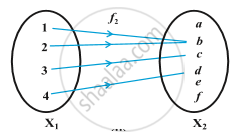Here, let's say "X"_1 is a set of all children and "X"_2 is a set of all womens. And "X"_1 and "X"_2 have connection as mother and children.
So as per the definition there is a connention between 2 sets "X"_1 and "X"_2 such that all the elements of "X"_1 are associated to some element in set "X"_2 i.e all the childrens are related to a particualr mother, and this association is unique because no one child can have two or more mothers, but a mother can have more than one child.
Examples-
X= {1,2,3,4}
Y= {1,5,,9,11,15,16}
Are the following relations functions?
a) "f"_1= {(1,1), (2,11), (3,1), (4,15)}
As per the definition this is the function.
b) "f"_2= {(1,1), (2,7), (3,5)}
This is not a function because 4 is not related with any element in set Y.
c) "f"_3= {(1,5), (2,9), (3,1), (4,5), (2,11)}
This is not a function because 2 is associated to 9&11.
This can also be written as "f"_3(2)= 9 and       "f"_3(2)= 11
i.e f(x)=y
(x,y)∈f
⦁ Domain of a Function- Domain is known as the input of a functin where all the values of x, for which f(x) stays defined or gives a valid answer.
Example- f(x)= x^3- 3
x∈R, so R is the domain here.
⦁ Range of a Function- It the ouput of a function. The range of a function is the complete set of all possible resulting values of the dependent variable (y, usually), after we have substituted the domain. The definition means the range is the resulting y-values we get after substituting all the possible x-values.
Example- f(x)= sqrt(x-1)
y= sqrt(x-1)
y^2= x-1
x= y^2+ 1
y∈R, so R is the range here.
⦁ Co-domain of a funcion- The codomain or target set of a function is the set Y into which all of the output of the function is constrained to fall. It is the set Y in the notation f: X → Y.
Types of functions-
1) One one (injective) function- If each element have single image, the it is a one one function.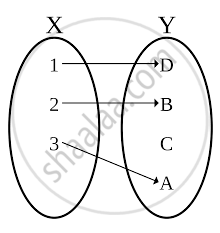Example- f(x)= 2x+3
if x=1, then y=5
x=2, y=7

x=1/2, y= 4  Here, every element of x have a separate value of y, thus it is a one one function.
One one function is further split into two parts
i) Onto one one function-  If each element of the codomain is mapped to by at least one element of the domain is an onto one one function. Co-domain= Range i.e y= f(x)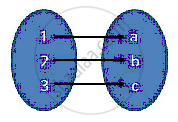ii)Into one one function- If each element of the codomain is not mapped to any one element of  the domain it is an into one one funcion.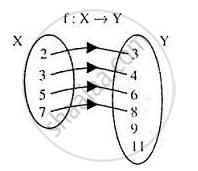2) Many one (surjective) function- In mathematics, a function f from a set X to a set Y is surjective, or a surjection, if for every element y in the codomain Y of f there is at least one element x in the domain X of f such that f(x) = y. It is not required that x be unique; the function f may map one or more elements of X to the same element of Y.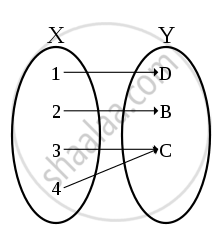Example- "f"(x)= x^2
if x=1, then y= 1
x= -1, y= 1
x=2, y= 4
x= -2, y= 4
Here, element of y have more than one values of x, thus it is a onto function.
Many one function is further split into two parts
i) Onto many one funcion- If two or more element of domain have one image and codomain is equal to range then it is said to be Onto many one function.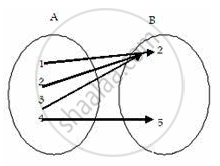ii) Into many one function- If two or more element of domain have one image and codomain is not equal to range then it is said to be Into many one function.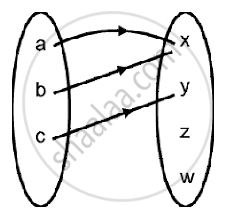If you would like to contribute notes or other learning material, please submit them using the button below.

### Shaalaa.com

Relations and Functions part 18 (function - Injective, one-one) [00:06:18]
S
0%